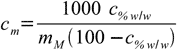# Concentration calculation

CASC concentration calculator

operating systems:
XP, Vista, 7, 8, 10

single user license price:
€49.95 - approximately \$65

30-day money back guarantee!

## Concentration lectures » percentage to molality conversion

Molality conversions are more demanding, as we need to separate solvent and solute. Let's start with definitions of both molality and mass percentage:11.111.2

At first sight there are no common points in both equations. For sure we will need additional information about molar mass of the substance.11.3

Combining 11.1 with 11.3 we get11.4

1000 factor is necessary as molality is expressed in [mole/kg] while all masses are in grams.

If we could rearrange our definition of weight percentage in such a way that that we will have msubstance/msolvent quotient on the left side of the equation, our conversion formula will be ready. Let's try. First, we multiply both sides of 11.2 by the msubstance+msolvent sum:11.5

Now let's group all terms containing both masses on both sides of equation:11.6

Almost done:11.7

And finally replacing msubstance/msolvent quotient in 11.4 by 11.7:11.8

If you solve this equation for c%w/w you will get formula for opposite conversion - from molality to weight percentage.